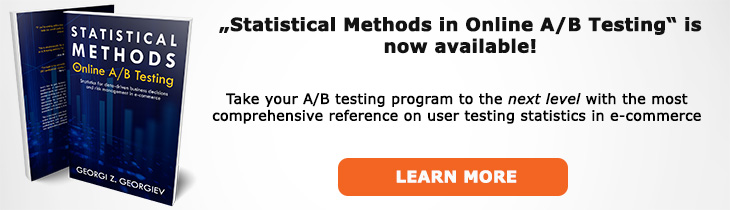# What does "One-Sided Hypothesis" mean?

Definition of One-Sided Hypothesis in the context of A/B testing (online controlled experiments).

## What is a One-Sided Hypothesis?

A one-sided hypothesis is an alternative hypothesis strictly bounded from above or from below, as opposed to a two-sided hypothesis which is the union of two one-sided hypotheses and is thus unbounded from both above and below. For example, H1: δ > 0 (alt.: H1: θ∈(0,+∞)) is a one-sided hypothesis since the parameter is bounded from below by zero. The corresponding null hypothesis would also be one-sided: H0: δ ≤ 0 (alt.:H0: θ∈(-∞,0])).

A one-sided alternative hypothesis is always used in a superiority test (most A/B tests) as well as in a non-inferiority test. As a statement it corresponds to the claim that the treatment will perform better than the control. While many examples set it to be less than or greater than zero, a one-sided hypothesis can be bounded at any value. For example, if the claim is that effect size is larger than 2% relative lift then H1: δ > 2%.

Consequently, a one-sided null hypothesis is always the complement of a one-sided alternative.

When a one-sided hypothesis is used the respective p-value should also be one-sided (or a one-tailed test as it is sometimes called). If a confidence interval is used to support a one-sided claim it should also be one-sided: one confidence limit is plus or minus infinity, depending on the direction of the hypothesis. If the distribution is symmetric around the mean and you can only calculate a one-sided p-value, a simple way to transform it is to divide it by to. E.g. a two-sided p-value of 0.1 is a one-sided p-value of 0.05. Similarly, a 95% two-sided confidence interval is composed of the intersection of two 97.5% one-sided confidence intervals.

## Articles on One-Sided Hypothesis

Like this glossary entry? For an in-depth and comprehensive reading on A/B testing stats, check out the book "Statistical Methods in Online A/B Testing" by the author of this glossary, Georgi Georgiev.## Glossary Index by Letter

Select a letter to see all A/B testing terms starting with that letter or visit the Glossary homepage to see all.